# Class and Object in Swift

## 1- Introduction

This document is based on:

• ### XCode 7.3

Swift is an inherited language from C and Objective-C, it is both a procedural language, both as a object oriented language. Class is a concept of the  object-oriented programming language. Class have properties and methods, naturally, method  is understood as a function of the class. From class you can create objects.
Swift using the class  keyword to declare a class.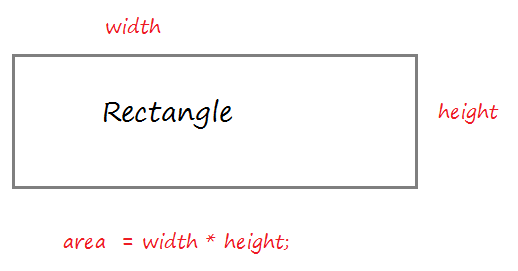Create a resource file Rectangle.swift:
Rectangle.swift
```import Foundation

class Rectangle  {

// width property
var width: Int = 5;

// height property
var height: Int = 10;

// Default Constructor (No parameter)
// (Used to create instance).
init()  {

}

// Contructor with 2 parameters.
// (Used to create instance)
// self.width refers to the width property of the class
init (width: Int, height: Int)  {
self.width = width
self.height = height

}

// A method calculates the area of the rectangle.
func getArea()  -> Int  {

var area = self.width * self.height
return area
}

}
```
The code using Rectangle class to create the  object.
RectangleTest.swift
```import Foundation

func test_rectangle()  {

print("Create a Rectangle rec1");

// Create Rectangle object
// via default constructor: init()
var rec1  = Rectangle()

// Print out width, height
print("rec1.width = \(rec1.width)")
print("rec1.height = \(rec1.height)")

// Call the method to calculate the area.
var area1 = rec1.getArea()

print("area1 = \(area1)")

print("---------")

print("Create a Rectangle rec2");

// Create Rectangle object
// via contructor with 2 parameters: init(Int,Int)
var rec2 = Rectangle(width: 10, height: 15)

// Print out width, height
print("rec2.width = \(rec2.width)")
print("rec2.height = \(rec2.height)")

// Call the method to calculate the area.
var area2 = rec2.getArea()

print("area2 = \(area2)")

}
```
Edit main.swift to test this example.
main.swift
```import Foundation

test_rectangle()

```
Running the example: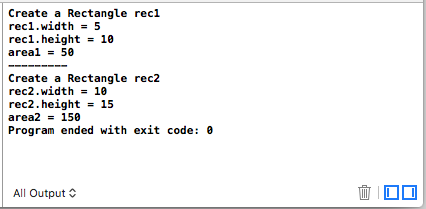Now you should see the explanation of the Class, it's very important.
What happens when you create an object by default contructor?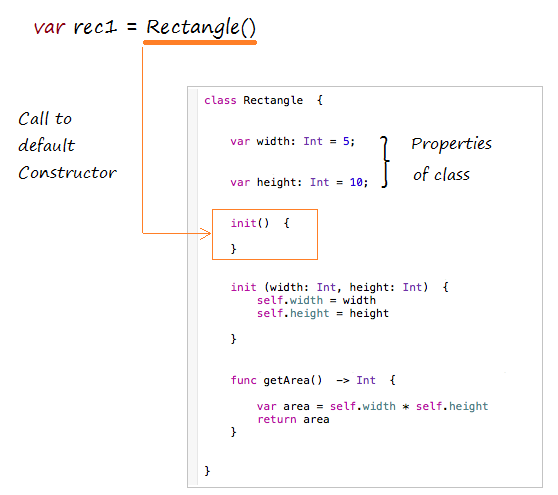Create a Rectangle object by contructor with 2 parameters.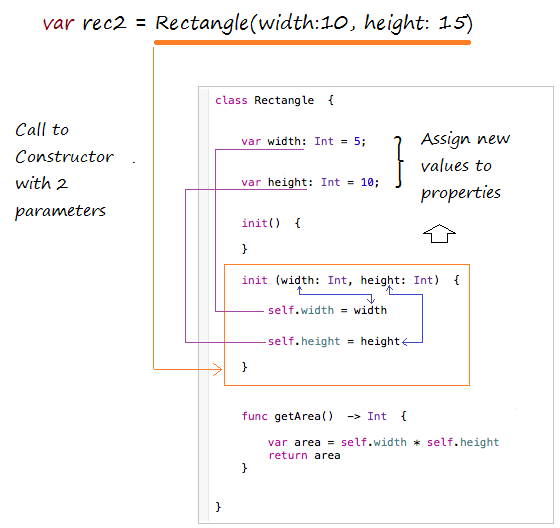## 3- Rule to call Constructor

Rules for Swift 2.1 to call function, method, constructor:
• First parameter pass to methods and functions should not have required argument labels.
• Other parameters pass to methods and functions should have required argument labels.
• All parameters pass to Constructors should have required argument labels.

## 4- Object Comparison operators

In Swift, when you create an object through Constructor will be a real entity is created in memory, it has a specified address.

An operator assigned AA object by an BB  object does not create new entity on the memory, it's just pointing to address of AA to BB's address.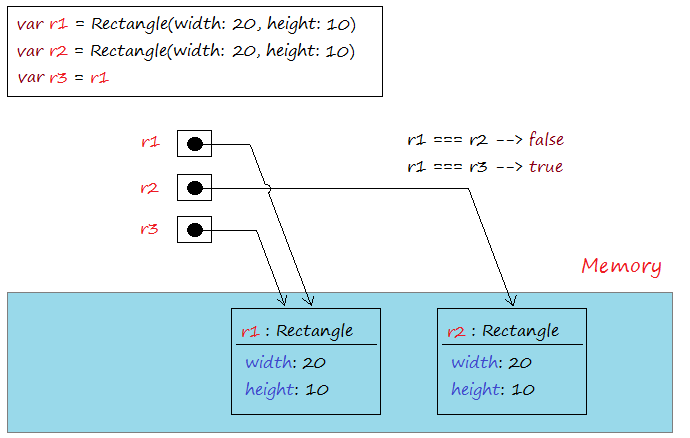=== Operator used to compare two objects that is pointing to, it returns true if the two objects refer to the same address in memory. Operators !== is used to compare the two addresses of two objects that is pointing to, it returns true if the two objects that point to two different addresses.
IdentifyOperator.swift
```import Foundation

func identifyOperator()    {

// Create object: r1
var r1 = Rectangle(width: 20,height: 10)

// Create object: r2
var r2 = Rectangle(width: 20,height: 10)

var r3 = r1

var  b12:Bool =   r1 === r2
var  b13:Bool =   r1 === r3

print("r1 === r2 ?  \(b12)") // false
print("r1 === r3 ?  \(b13)") // true

var bb12: Bool = r1 !== r2
var bb13: Bool = r1 !== r3

print("r1 !== r2 ?  \(b12)") // true
print("r1 !== r3 ?  \(b13)") // false

}
```
Running the example: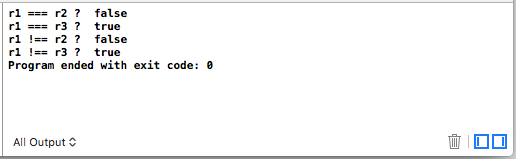#### View more Tutorials:

Maybe you are interested

These are online courses outside the o7planning website that we introduced, which may include free or discounted courses.

•*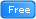* Basic Swift 4 and iOS 11 - Free Preview
•Make iPhone Apps Using Swift, Xcode and iOS8 - 7 Apps
•iOS 11 Programming with SWIFT
•iOS Development - Create 4 Quiz Apps with Swift 3 & iOS 10
•Swift Language Fundamentals
•iOS 11 / Swift 4 - Programming 101
•Create an app like Twitter with Swift
•Beginner's Swift 4 & Unreal Engine - Learn Xcode, Make Games
•Swift - Advanced API's & Technique
•C# Unity® and Swift 4 Masterclass: Make Games and Apps
•iOS 11 & Swift 4.0 - A Beginner's Guide with Projects
•iOS 10 Swift 3 - A Complete Practical Approach
•iOS 11 & Swift 4: From Beginner to Paid Professional™
•** Swift for Beginners, Learn Apple's New Programming Language
•Swift - Learn by doing